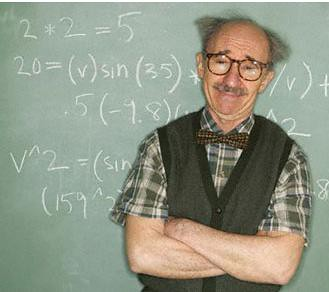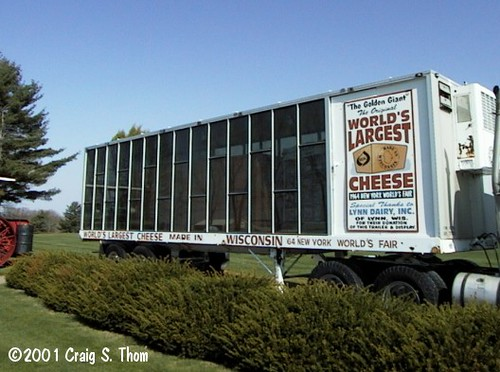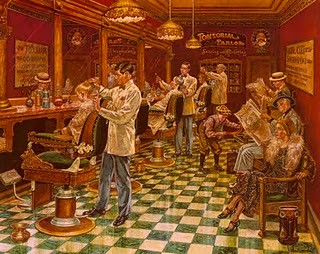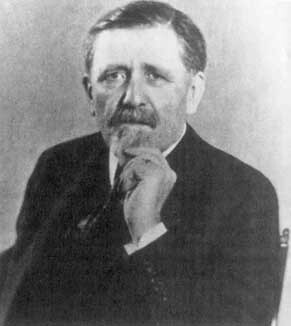(Roughly) Daily

Fun with numbers!…

Gary Foshee, a collector and designer of puzzles from Issaquah near Seattle walked to the lectern to present his talk. It consisted of the following three sentences: “I have two children. One is a boy born on a Tuesday. What is the probability I have two boys?”

The event was the Gathering for Gardner [see here], a convention held every two years in Atlanta, Georgia, uniting mathematicians, magicians and puzzle enthusiasts. The audience was silent as they pondered the question.

“The first thing you think is ‘What has Tuesday got to do with it?'” said Foshee, deadpan. “Well, it has everything to do with it.” And then he stepped down from the stage.

Read the full story of the conclave– held in honor of the remarkable Martin Gardner, who passed away last year, and in the spirit of his legendary “Mathematical Games” column in Scientific American— in New Scientist…  and find the answer to Gary’s puzzle there– or after the smiling professor below.“I have two children. One is a boy born on a Tuesday. What is the probability I have two boys?”…  readers may hear a Bayesian echo of the Monty Hall Problem on which (R)D has mused before:

The first thing to remember about probability questions is that everyone finds them mind-bending, even mathematicians. The next step is to try to answer a similar but simpler question so that we can isolate what the question is really asking.

So, consider this preliminary question: “I have two children. One of them is a boy. What is the probability I have two boys?”

This is a much easier question: The way Foshee meant it is, of all the families with one boy and exactly one other child, what proportion of those families have two boys?

To answer the question you need to first look at all the equally likely combinations of two children it is possible to have: BG, GB, BB or GG. The question states that one child is a boy. So we can eliminate the GG, leaving us with just three options: BG, GB and BB. One out of these three scenarios is BB, so the probability of the two boys is 1/3.

Now we can repeat this technique for the original question. Let’s list the equally likely possibilities of children, together with the days of the week they are born in. Let’s call a boy born on a Tuesday a BTu. Our possible situations are:

* When the first child is a BTu and the second is a girl born on any day of the week: there are seven different possibilities.
* When the first child is a girl born on any day of the week and the second is a BTu: again, there are seven different possibilities.
* When the first child is a BTu and the second is a boy born on any day of the week: again there are seven different possibilities.
* Finally, there is the situation in which the first child is a boy born on any day of the week and the second child is a BTu – and this is where it gets interesting.

There are seven different possibilities here too, but one of them – when both boys are born on a Tuesday – has already been counted when we considered the first to be a BTu and the second on any day of the week. So, since we are counting equally likely possibilities, we can only find an extra six possibilities here.

Summing up the totals, there are 7 + 7 + 7 + 6 = 27 different equally likely combinations of children with specified gender and birth day, and 13 of these combinations are two boys. So the answer is 13/27, which is very different from 1/3.

It seems remarkable that the probability of having two boys changes from 1/3 to 13/27 when the birth day of one boy is stated – yet it does, and it’s quite a generous difference at that. In fact, if you repeat the question but specify a trait rarer than 1/7 (the chance of being born on a Tuesday), the closer the probability will approach 1/2.

[See UPDATE, below]

As we remember, with Laplace, that “the theory of probabilities is at bottom nothing but common sense reduced to calculus,” we might ask ourselves what the odds are that on this date in 1964 the World’s Largest Cheese would be manufactured for display in the Wisconsin Pavilion at the 1964-65 World’s Fair.  The 14 1/2′ x 6 1/2′ x 5 1/2′, 17-ton cheddar original– the product of 170,000 quarts of milk from 16,000 cows– was cut and eaten in 1965; but a replica was created and put on display near Neillsville, Wisconsin… next to Chatty Belle, the World’s Largest Talking Cow.The replica on display (source)

UPDATE: reader Jeff Jordan writes with a critique of the reasoning used above to solve Gary Foshee’s puzzle:

For some reason, mathematicians and non-mathematicians alike develop blind
answer, and are trying to convince others of its correctness. While I agree
with most of your analysis, it has one such blind spot. I’m going move
through a progression of variations on another famous conundrum, trying to
isolate these blind spots and eventually get the point you overlooked.

Bertrand’s Box Paradox: Three identical boxes each have two coins inside:
one has two gold coins, one has two silver coins, and one has a silver coin
and a gold coin. You open one and pull out a coin at random, without seeing
the other. It is gold. What is the probability the other coin is the same
kind?

A first approach is to say there were three possible boxes you could pick,
but the information you have rules one out. That leaves two that are still
possible. Since you were equally likely to pick either one before picking a
coin, the probability that this box is GG is 1/2. A second approach is that
there were six coins that were equally likely, and three were gold. But two
of them would have come out of the GG box. Since all three were equally
likely, the probability that this box is GG is 2/3.

This appears to be a true paradox because the “same” theoretical approach –
counting equally likely cases – gives different answers. The resolution of
that paradox – and the first blind spot – is that this is an incorrect
theoretical approach to solving the problem. You never want to merely count
cases, you want to sum the probabilities that each case would produce the
observed result. Counting only works when each case that remains possible
has the same chance of producing the observed result. That is true when you
count the coins, but not when you count the boxes. The probability of
producing a gold coin from the GG box is 1, from the SS box is 0, and from
the GS box is 1/2. The correct answer is 1/(1+0+1/2)=2/3. (A second blind
spot is that you don’t “throw out” the impossible cases, you assign them a
probability of zero. That may seem like a trivial distinction, but it helps
to understand what probabilities other than 1 or 0 mean.)

This problem is mathematically equivalent to the original Monty Hall
Problem: You pick Door #1 hoping for the prize, but before opening it the
host opens Door #3 to show that it is empty. Given the chance, what is the
probability you win by switching to door #2? Let D1, D2, and D3 represent
where the prize is. Assuming the host won’t open your door, and knows where
the prize is so he always opens an empty door, then the probability D2 would
produce the observed result is 1, that D3 would is 0, and that D1 is …
well, let’s say it is 1/2. Just like before, the probability D2 now has the
prize is 1/(1+0+1/2)=2/3.

Why did I waffle about the value of P(D1)? There was a physical difference
with the boxes that produced the explicit result P(GS)=1/2. But here the
difference is logical (based on the location of the prize) and implicit. Do
we really know the host would choose randomly? In fact, if the host always
opens Door #3 if he can, then P(D1)=1 and the answer is 1/(1+0+1)=1/2. Or if
he always opens Door #2 if he can, P(D1)=0 and the answer is 1/(1+0+0)=1.
But if we observe that the host opened Door #2 and assume those same biases,
the results reverse.

To answer the question, we must assume a value for P(D1). Assuming anything
other than P(D1)=1/2 implies a bias on the part of the host, and a different
answer if he opens Door #2. So all we can assume is P(D1)=1/2, and the
answer is again 2/3. That is also the answer if we average the results over
many games with the same host (and a consistent bias, whatever it is). The
answer most “experts” give is really that average, and it is a blind spot
that they are not using all the information they have in the individual
case.

We can make the Box Paradox equivalent to this one by making the random
selection implicit. Someone looks in the chosen box, and picks out a gold
coin. The probability is 2/3 that there is another gold coin if that person
picks randomly, 1/2 if that person always prefers a gold coin, and 1 if that
person always prefers a silver one. Without knowing the preference, we can
only assume this person is unbiased and answer 2/3. Over many experiments,
it will also average out to 2/3 regardless of the bias. And this person
doesn’t even have to show the coin. If we assume he is truthful (and we can
only assume that), the answers are the same if he just says “One coin is
gold.”

Finally, make a few minor changes to the Box Paradox. Change “silver” to
“bronze.” Let the coins be minted in different years, so that the year
embossed on them is never the same for any two. Add a fourth box so that one
box has an older bronze coin with a younger gold coin, and one has a younger
bronze coin with an older gold coin. Now we can call the boxes BB, BG, GB,
and GG based on this ordering. When our someone says “One coin is bronze,”
we can only assume he is unbiased in picking what kind of coin to name, and
the best answer is 1/(1+1/2+1/2+0)=1/2. If there is a bias, it could be
1/(1+1+1+0)=1/3 or 1/(1+0+0+0)=1, but we can’t assume that. Gee, this sounds
oddly familiar, except for the answer. :)

The answer to all of Gary Foshee’s questions is 1/2. His blind spot is that
he doesn’t define events, he counts cases. An event a set of outcomes, not
an outcome itself. The sample space is the set of all possible outcomes. An
event X must be defined by some property such that every outcome in X has
that property, *and* every outcome with the property is in X. The event he
should use as a condition is not “this family includes a boy (born on a
Tuesday)”, it is “The father of this family chooses to tell you one of up to
two facts in the form ‘my family includes a [gender] (born on a [day]).'”
Since most fathers of two will have two different facts of that form to
choose from, Gary Foshee should have assigned a probability to each, not
merely counted the families that fit the description. The answer is then
(1+12P)/(1+26P), where P is the probability he would tell us “one is a boy
born on a Tuesday” when only one of his two children fit that description.
The only value we can assume for P is 1/2, making the answer
(1+6)/(1+13)=1/2. Not P=1 and (1+12)/(1+26)=13/27.

And the blind spot that almost all experts share, is that this means the
answer to most expressions of the simpler Two Child Problem is also 1/2. It
can be different, but only if the problem statement makes two or three
points explicit:

1) Whatever process led to your knowledge of one child’s gender had access
to both children’s genders (and days of birth).
2) That process was predisposed to mention boys over girls (and Tuesdays
over any other day).
3) That process would never mention facts about both children.

When Gary Foshee tells you about one of his kids, #2 is not satisfied. He
probably had a choice of two facts to tell you, and we can’t assume he was
biased towards “boy born on Tuesday.” Just like Monty Hall’s being able to
choose two doors changes the answer from 1/2 to 2/3, Gary Foshee’s being
able to choose two facts changes the answer from 13/27 to 1/2. It is only
13/27 if he was forced to mention that fact, which is why that answer is
unintuitive.

Other readers are invited to contribute their thoughts.

Written by (Roughly) Daily

January 22, 2011 at 1:01 amSuppose there is a town with just one male barber; and that every man in the town keeps himself clean-shaven: some by shaving themselves, some by attending the barber. It seems reasonable to imagine that the barber obeys the following rule: He shaves all and only those men in town who do not shave themselves. Under this scenario, we can ask the following question: Does the barber shave himself?Borel (image source)